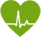#### Calorie Budget

The Calorie Calculator can be used to estimate the calories you should consume each day. This calculator can also provide a baseline if you want to lose or gain weight.

0
##### Basal Metabolic Rate (BMR)

The number of calories that the body needs to support vital functions if, hypothetically, a person were resting in bed for 24 hours is known as the basal metabolic rate, or BMR. The daily calorie budget listed above is calculated using the Harris Benedict Formula. In order to use this formula, a person would need to know his or her BMR, calculated using the following equation:

• Women

BMR = 655 + ( 9.6 x weight in kilos ) + ( 1.8 x height in cm ) - ( 4.7 x age in years )
• Men

BMR = 66 + ( 13.7 x weight in kilos ) + ( 5 x height in cm ) - ( 6.8 x age in years )